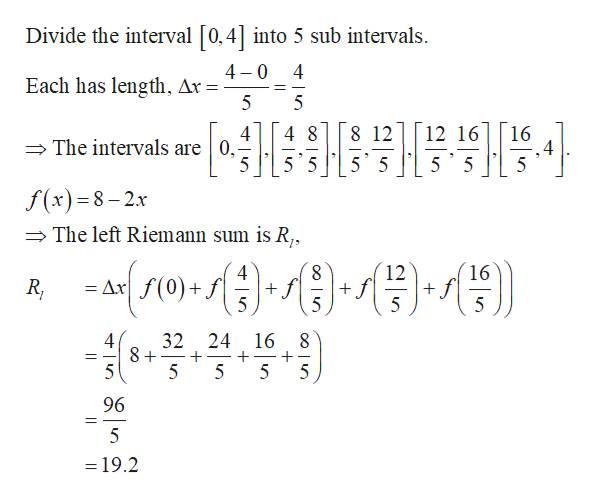# Let f(x) = 8 - 2x (a) Use a Riemann sum with five subintervals of equal length (n = 5) to approximate the area of R. Choose the representative points to be the left endpoints of the subintervals. (b) Repeat part (a) with ten subintervals of equal length (n = 10).

Question
51 views

Let f(x) = 8 - 2x

(a) Use a Riemann sum with five subintervals of equal length (n = 5) to approximate the area of R. Choose the representative points to be the left endpoints of the subintervals.

(b) Repeat part (a) with ten subintervals of equal length (n = 10).

check_circle

Step 1

(a) Use a Riemann sum with 5 sub intervals to approximate the area of R...help_outlineImage TranscriptioncloseDivide the interval [0,4] into 5 sub intervals 4 0 Each has length, Ax = 5 4 5 8 12 16 4 5 4 The intervals are 0,- 48 12 16 5 5 5 5 5 5 f(x)8-2x The left Riemann sum is R, 16 12 f f 5 4 8 Ax f(0)+f 5 5 5 24 16 4 32 8+ 5 5 5 5 96 = 19.2 fullscreen

### Want to see the full answer?

See Solution

#### Want to see this answer and more?

Solutions are written by subject experts who are available 24/7. Questions are typically answered within 1 hour.*

See Solution
*Response times may vary by subject and question.
Tagged in

### Other